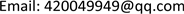HJCEHans Journal of Civil Engineering2326-3458Scientific Research Publishing10.12677/HJCE.2018.74064HJCE-25251HJCE20180400000_94597691.pdf工程技术 水泥砼桥面沥青铺装受力影响研究 Study on the Influence of Force on Cement Asphalt Pavement Asphalt Pavement 彦昌21红涛3*41长沙理工大学，湖南 长沙漯河市公路管理局，河南 漯河漯河市公路工程建设总公司，河南 漯河null* E-mail:420049949@qq.com(李红);010620180704559566© Copyright 2014 by authors and Scientific Research Publishing Inc. 2014This work is licensed under the Creative Commons Attribution International License (CC BY). http://creativecommons.org/licenses/by/4.0/

1漯河市公路管理局，河南 漯河

2漯河市公路工程建设总公司，河南 漯河

3长沙理工大学，湖南 长沙Copyright © 2018 by authors and Hans Publishers Inc.1. 引言

2. 有限元模型的建立

Bridge deck pavement system material parameter

3. 行车状况对沥青铺装体系受力的影响

3.1. 车辆超载对沥青铺装体系受力的影响

( P i P 0 ) 0.65 = p i p 0 (2.1)

p i ——超载车辆轮胎接地压强(MPa)；

P 0 ——标准轴载(kN)；

p 0 ——标准轴载轮胎接地压力(MPa)。

P i = p i s (2.2)

Different paving structure composite material parameter

Load calculation parameters corresponding to different overload rate

01000.91109.8925
201201.02117.6530
401401.13123.8931
601601.23130.0833
801801.33135.3434
1002001.43139.8635

σ M A X = 0.0032 X + 0.2430         R 2 = 0.9901 (2.3)

τ M A X = 0.0020 X + 0.1860         R 2 = 0.9898 (2.4)

σ M A X = 0.0012 X + 0.0898         R 2 = 0.9936 (2.5)

τ M A X = 0.0021 X + 0.1937         R 2 = 0.9914 (2.6)

σ M A X = 0.0021 X + 0.1625         R 2 = 0.9871 (2.7)

τ M A X = 0.0020 X + 0.1864         R 2 = 0.9905 (2.8)

3.2. 车辆刹车对沥青铺装体系受力的影响

F = f P (2.9)

P——车辆垂直荷载(kN)；

f——水平摩阻系数。

σ M A X = 2.1524 f 2 − 0.2169 f + 0.2247         R 2 = 0.9927 (2.10)

τ M A X = 0.3095 f + 0.1737         R 2 = 0.9996 (2.11)

σ M A X = 1.9440 f 2 − 0.0887 f + 0.1433         R 2 = 0.9889 (2.12)

τ M A X = 0.3125 f + 0.1816         R 2 = 0.9993 (2.13)

σ M A X = 1.7127 f 2 + 0.0535 f + 0.0761         R 2 = 0.9871 (2.14)

τ M A X = 0.3108 f + 0.1742         R 2 = 0.9995 (2.15)

4. 温度变化对沥青铺装体系受力的影响

4.1. 温度传递基本理论和边界条件

q ( x , y , z , t ) = − k Δ T ( x , y , z , t ) (3.1)

∆T——温度梯度(℃/m)；

k——导热系数(W/m℃)；

t——作用时间(s)。

q ( x , y , z , t ) = − o k ∂ T ∂ x − p k ∂ T ∂ y − q k ∂ T ∂ z (3.2)

q x = − k ∂ T ∂ x q y = − k ∂ T ∂ y q z = − k ∂ T ∂ z (3.3)

Δ 2 T ( x , y , z , t ) = 1 α ∂ T ( x , y , z , t ) ∂ t (3.4)

∂ 2 T ∂ x 2 + ∂ 2 T ∂ y 2 + ∂ 2 T ∂ z 2 = 1 α ∂ T ∂ t (3.5)

T = f i ( x , y , z , t ) (3.6)

4.2. 温度应力分析

σ M A X = 0.0005 T 2 + 0.0440 T + 0.0262         R 2 = 0.9987 (3.7)

τ M A X = 0.0008 T 2 − 0.0027 T + 0.1800         R 2 = 0.9919 (3.8)

σ M A X = 0.0002 T 2 + 0.0355 T + 0.0750         R 2 = 0.9981 (3.9)

τ M A X = 0.0008 T 2 − 0.0096 T + 0.2031         R 2 = 0.9906 (3.10)

σ M A X = 0.0003 T 2 + 0.0336 T + 0.0810         R 2 = 0.9979 (3.11)

τ M A X = 0.0008 T 2 − 0.0071 T + 0.1899         R 2 = 0.9866 (3.12)

5. 结论

1) 超载作用对铺装层顶影响较大，应严格控制超载现象的发生；

2) 当水平摩阻系数超过0.2之后，层顶最大拉应力迅速增加；而界面最大剪应力与水平摩阻系数呈较好的线性相关，并且三种方案相差较小；

3) 各方案层顶最大拉应力随着温度减低而增大，都呈较好的线性关系，这也表明了在低温季节铺装结构顶面承受着较大拉应力，一旦超过铺装材料强度容许值，就会产生开裂。